# Analysis of math function based controller for a smooth transition between battery and ultracapacitor

Analysis of math function based controller for a smooth transition between battery and ultracapacitor

Raghavaiah KaturiSrinivasarao Gorantla

Department of Electrical and Electronics Engineering, Vignan’s Foundation for Science, Technology, and Research, Vadlamudi, Guntur 522213, Andhra Pradesh, India

Corresponding Author Email:
rk_eeep@vignanuniversity.org
Page:
386-394
|
DOI:
https://doi.org/10.18280/mmep.050416
22 October 2018
|
Accepted:
1 December 2018
|
Published:
31 December 2018
| Citation

OPEN ACCESS

Abstract:

To obtain automatic smooth switching between energy sources present in Hybrid Energy Storage System (HESS) is the main problem associated with Hybrid Electric Vehicles / Electric Vehicles (HEVs/EVs). The key objective of this work is to design a particular control strategy which is useful to switch the battery and ultracapacitor (UC) corresponding to the vehicle dynamics.  In this work a new control strategy is realized by hybridizing two controllers. A math function based controller (MFB) is designed with four separate math functions in association with speed of an electric motor, which indicates that the speed of an electric motor plays a vital role during transition of energy sources. The combination, MFB and conventional Proportional-Integral-Derivative (PID) controller forms the hybrid controller which meets the main objective of the proposed work. Finally, the designed hybrid controller generates and regulates the switching signal of DC- DC converters with smooth transition can be obtains corresponding to the vehicle dynamics. The proposed methodology is implemented especially in four modes with different load; all simulation results are plotted and discussed.

Keywords:

electric vehicles (EVs), battery, ultracapacitor (UC), bidirectional converter, unidirectional converter, math function based (MFB) controller, proportional-integral-derivative (PID) controller

1. Introduction

Most of EVs/HEVs are fed with a conventional fuel source for its successful operation. In that, some of the pros and cons are identified and resolved those things up to the maximum extent. Hybridization of the base source can be achieved with another alternate source to achieve better objective from EVs/HEVs. Generally, all base sources are capable of delivering energy with high energy density, on the other hand supporting sources like UC is capable of delivering power with high power density. By combining base source with supporting one forms a new HESS, which will enhance the performances of the particular vehicle during all load conditions [1-10].

Anciently Battery is used to propel the electric vehicle in all road conditions like starting, running and transient period of the vehicle. A hybrid flywheel/battery system is used for storing the braking energy and it can be utilized during peak loads of electric vehicles in order to reduce the burden on the battery . The embedded energy storage system has been made with Fuel cell and UC, a polynomial method is used to split the energy between two sources according to the energetic request of HEV. A fuel cell is used for meeting the average power and UC is used for fluctuating for the requirement of HEV . The energy management between high energy density device battery and high power density device UC has been made with the controller for emergency starting of an IC engine to save the life of the rich energy device .In the designed power source UC gets charged from the battery very quickly and discharges energy at the same rate which makes the IC engine proper starting .

The HESS has been developed for EV/HEV application in that, energy management is the main issue to split the energy between battery and UC. Energy management strategy has developed with an adaptive fuzzy logic controller to manage the power splitting between the two sources. The designed adaptive fuzzy logic controller enhances the overall system efficiency by sharing the transient power to the UC and average power to the battery .To make proper energy management between the battery and UC 2- real-time controllers have been developed for the finest current sharing between UC and battery in an EV application. One of the controllers is developed based on Karush–Kuhn– Tucker conditions by solving the formulated optimization problem, which makes the right current splitting of HESS. The second controller is developed based on neural network and that is termed as an intelligent controller. Based on the current sharing of energy sources controller performance has been evaluated . With a variable rate –limit function an adaptive energy management control has been designed for the energy storage system. The designed energy sharing controller saving the life of the main source of the energy storage system (ESS). Steady state power can be supplied by the main source and aggressive power during the transient period can be supplied by the auxiliary source. Here the auxiliary source is capable of charging and discharging of energy at very less time though it has low energy density property .

An effective energy management scheme is designed with the neural network system. The HEV fed with various primary sources like a fuel cell, a battery which is unable to grab the energy during the regenerating period of the vehicle. In order to store energy during regenerating breaking effectively, UC banks are used. In order to store and measure the instantaneous current, vehicle speed and main source voltage values, the digital signal processor is used. Initially, energy management can be done in a conventional way thereafter used a neural network approach to obtain better performance . HESS has been implemented with UC and battery and the bidirectional DC-DC converter is used with low power for the design of the controller for proper power management of the input sources. The HESS is operated in four modes within its power limit for proper power split between UC and battery . Energy management strategy (EMS) plays an important role in efficient HEVs. General two types of EMS are used for optimal power splitting in that first one is algorithm based EMS and the second one is the rule-based approach for easy analysis. Hybrid EMS has been designed for utilizing the advantages from two EMS approaches. To obtain the effective energy management of HEV different DC-DC converter topologies are adopted. The supercapacitor (SCAP) bank is integrated into the already existed test bench system. The test bench system mainly containing with two motors and those are separately connected to the alternators to generate the required power to the load. The main intention of this work is to provide peak power with SCAP with a short interval of the time period that may be 20 sec. The original system consisting with main power source only and in this additionally, supper capacitor is connected to enhance the overall performance of the system. . EMS has been implemented based on the numerical methods for HEVs. This controller does not need the previous driving data and the overall controller can be achieved according to the driver requirements and is formulated as nonlinear receding horizon control (RHC) problem .

In this work, an altered control scheme is proposed to develop the controlled switching signals to the switches present in BDC as well as UDC based on the load applied to the motor. The designed strategy mainly works corresponding to the speed of the electric motor with this proper smooth transition can be made between the energy source of HESS. This work organized mainly with eight chapters. In chapter two proposed system model is projected, the mathematical modeling of HESS is done in chapter three. Description of controllers used in the proposed control strategy is discussed in chapter four , In  chapter five modes of operation  of the converter model is discussed, the proposed control strategy is explained in chapter  six. Simulation results and discussions are presented in chapter seven and finally, conclusions are discussed in chapter eight.

2. Proposed System Model

The proposed system model mainly comprising with two controllers named as conventional PID as well as MFB controller. And the energy sources battery and UC, here battery is treated as a base source on the other hand UC treated as supporting source. The UC will support the main source during extreme conditions like stating as well as more than rated load condition and which also gets charged during no load condition by taking energy from the battery.

Figure 2 shows the main circuit model with DC-DC converters in which unidirectional converter (UDC) and Bidirectional converter (BDC) are connected at the battery as well as UC end. Here BDC comprises with two switches named as S3 and S2 on the other hand UDC consisting of the only switch named as S1. And BDC will perform both boost as well as buck operation, and UDC performs only boost operation. This means UC capacitor will deliver the power to the motor during transient as well as stating condition of the motor and gets charged from the battery during no-load condition on the electric motor.

## 11.jpgFigure 1. The block diagram model of HESS with proposed control strategy approach

## 22.jpgFigure 2. Main circuit model with DC-DC converters

3. Mathematical Modelling of Hybrid Energy Storage System

3.1 Battery model

Figure 3 shows that the dynamical model of the battery, where the terminal voltage is a function of time and is finding from three components.

## 3.jpgFigure 3. A dynamical model of a battery

$\mathrm{f}_{1}\left(\mathrm{V}_{\mathrm{t}}, \mathrm{i}_{1}, \mathrm{i}\right)=\mathrm{E}_{0}-\frac{\mathrm{KQi}_{1}}{\mathrm{Q}-\mathrm{V}_{\mathrm{t}}}-\frac{\mathrm{KQV}_{\mathrm{t}}}{\mathrm{Q}-\mathrm{V}_{\mathrm{t}}}+\mathrm{E} . \exp \left(-\mathrm{D} . \mathrm{V}_{\mathrm{t}}\right)$ (1)

$\mathrm{f}_{1}\left(\mathrm{V}_{\mathrm{t}}, \mathrm{i}_{\mathrm{l}}, \mathrm{i}\right)=\mathrm{E}_{0}-\frac{\mathrm{K} \mathrm{Qi}_{1}}{\mathrm{V}_{\mathrm{t}}+0.1 \mathrm{Q}}-\frac{\mathrm{KQV}_{\mathrm{t}}}{\mathrm{Q}-\mathrm{V}_{\mathrm{t}}}+\mathrm{E} . \exp \left(-\mathrm{D} \cdot \mathrm{V}_{\mathrm{t}}\right)$ (2)

where E0=constant voltage (V)

$\mathrm{SOC}=100\left(1-\frac{1}{\mathrm{Q}} \int_{0}^{\mathrm{t}} \mathrm{i}(\mathrm{t}) \mathrm{d} \mathrm{t}\right)$ (3)

i=battery current

Q=Capacity of the battery (Ah)

Vt=Extracted voltage (V)

E=Exponential voltage (V)

I1=Low frequency current dynamics (A)

K=Polarization resistance (Ohms)

D=Exponential capacity (Ah-1)

3.2 Ultracapacitor model

## 4.jpg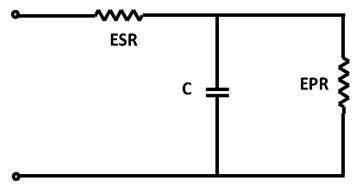Figure 4. The equivalent electrical model of UC

Figure 4 representing that the equivalent electrical model of UC. The voltage state of UC for RC is given by stern-Tafel model of the UC is the most popular model and the equations of this model can be written as

$\mathrm{V}=\frac{\mathrm{NN}_{\mathrm{s}} \mathrm{Q} \mathrm{X} 2}{\mathrm{NN}_{\mathrm{p}} \mathrm{N}^{\wedge} 2 \varepsilon \varepsilon 0 \mathrm{A}}+\frac{\mathrm{NN}_{\mathrm{s}} 2 \mathrm{RT}}{\mathrm{F}} \alpha . \mathrm{r} \cdot \sinh \left(\frac{\mathrm{Q}}{\mathrm{N}_{\mathrm{p}} \mathrm{N}^{2 \sqrt{8 \mathrm{RT} \varepsilon \varepsilon 0 \mathrm{c}}}}\right)$ (4)

$-\mathrm{i}(\mathrm{t})=\mathrm{Ai}_{0} \exp \left(\frac{\alpha \mathrm{F}\left(\frac{\mathrm{V}}{\mathrm{N}_{\mathrm{S}}}-\frac{\mathrm{V}_{\max }}{\mathrm{N}_{\mathrm{S}}}-\Delta \mathrm{V}\right)}{\mathrm{RT}}\right)$ (5)

$\mathrm{SOC}=\frac{\text { Qinit- } \int_{0}^{\mathrm{t}}(\mathrm{t}) \mathrm{d} \tau}{\mathrm{QT}} \times 100$ (6)

A= space between electrodes and electrolyte (m2)

F = Faraday constant, i = Current concentration (Am−2)

k =Stefan-Boltzmann constant

N = Number of layers of electrodes

Np = Parallel connected UCs

Ns = Series connected UCs, Q = Electric charge (C),

R= constant of ideal gas,

i0 = Exchange current density, i0 = if/A (Am−2)

ΔV = Change in voltage, α = Charge transfer coefficient,

ε = Material permittivity, ε0 = Free space permittivity

C = molar density (mol m−3) equal to c = 0.86/ (8NAr3

3.3 Unidirectional converter modeling

Figure 5 represents DC-DC Converter (boost), and the state space expression for the DC-DC converter (boost) during switch is in ON condition.

## 5.jpg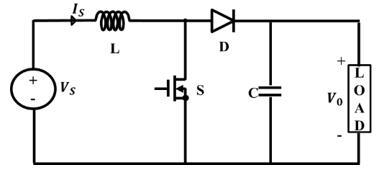Figure 5. Unidirectional converter model

The generalized equation of UDC converter can be written as;

$\mathrm{V}=\mathrm{L} \frac{\mathrm{di}}{\mathrm{dt}}$ (7)

$\mathrm{i}=\frac{1}{\mathrm{L}} \int_{0}^{\mathrm{t}} \mathrm{Vdt}+\mathrm{i}_{0}$ (8)

If the switch is in ON condition

$\mathrm{i}_{\mathrm{pk}}=\frac{\left(\mathrm{V}_{\mathrm{O}}-\mathrm{V}_{\mathrm{i}}\right) \mathrm{T}_{\mathrm{on}}}{\mathrm{L}}$ (9)

$\Delta \mathrm{i}=\frac{\left(\mathrm{V}_{\mathrm{O}}-\mathrm{VT}_{\mathrm{mos}}\right) \mathrm{T}_{\mathrm{on}}}{\mathrm{L}}$ (10)

If the switch is in OFF condition

$\mathrm{i}_{0}=\mathrm{i}_{\mathrm{pk}}=\frac{\left(\mathrm{V}_{\mathrm{O}}-\mathrm{V}_{\mathrm{i}}+\mathrm{V}_{\mathrm{D}}\right) \mathrm{T}_{\mathrm{off}}}{\mathrm{L}}$ (11)

or

$\Delta i=\frac{\left(V_{0}-V_{i}+V_{D}\right) T_{o f f}}{L}$ (12)

VD=Diode across voltage drop, Vmos  = Voltage drop across MOSFET

To find the V0 value equate the $\Delta \mathbf{i}$   then,

$V_{0}=\frac{V_{i}-V_{m o s} * \delta}{1-\delta}-V_{D}$ (13)

After neglecting the losses at MOSFET and Diode, the above equation becomes

$\mathrm{V}_{\mathrm{o}}=\frac{\mathrm{V}_{\mathrm{i}}}{1-\delta}$ (14)

Using the above equation (14) input voltage of the converter step up to a required voltage level by changing the duty cycle.

3.4 Bidirectional converter modeling

## 6.jpgFigure 6. Bidirectional converter model

From that BDC model inductor current can be written as;

$\frac{\mathrm{d} \mathrm{I}_{\mathrm{L}}}{\mathrm{dt}}=\frac{\mathrm{V}_{\mathrm{i}}}{\mathrm{L}}$ (15)

Change in inductor current is given by,

$\Delta \mathrm{I}_{\mathrm{Lon}}=\int_{0}^{\delta \mathrm{T}} \mathrm{d} \mathrm{IL}=\int_{0}^{\delta \mathrm{T}} \frac{\mathrm{V}_{\mathrm{i}}}{\mathrm{L}} \mathrm{dt}=\frac{\mathrm{V}_{\mathrm{i}} \delta \mathrm{T}}{\mathrm{L}}$ (16)

The equation for IL can be written as;

$\frac{\mathrm{d} \mathrm{IL}}{\mathrm{dt}}=\frac{\mathrm{V}_{0}}{\mathrm{L}}$ (17)

Therefore, the variation of IL

in the off-period is,

$\Delta \mathrm{I}_{\mathrm{Loff}}=\int_{0}^{(1-\delta) \mathrm{T}} \mathrm{dIL}=\int_{0}^{(1-\delta) \mathrm{T}} \frac{\mathrm{V}_{0}}{\mathrm{L}} \mathrm{dt}=\frac{\mathrm{V}_{0}(1-\delta) \mathrm{T}}{\mathrm{L}}$ (18)

It is obvious that the sum of variations in IL for on-state and off-state should be zero. Hence

$\Delta \mathrm{I}_{\mathrm{Lon}}+\Delta \mathrm{I}_{\mathrm{Loff}}=0$ (19)

Substituting the equations of $\Delta \mathrm{I}_{\text { Lon }}$ and $\Delta \mathrm{I}_{\text { Loff }}$

$\Delta \mathrm{I}_{\mathrm{Lon}}+\Delta \mathrm{I}_{\mathrm{Loff}}=\frac{\mathrm{V}_{\mathrm{i}} \delta \mathrm{T}}{\mathrm{L}}+\frac{\mathrm{V}_{0}(1-\delta) \mathrm{T}}{\mathrm{L}}=0$ (20)

This can be written as,

$\frac{V_{0}}{V_{i}}=\frac{\delta}{\delta-1}$ (21)

And

$\delta=\frac{V_{0}}{V_{0}-V_{i}}$ (22)

4. Description of Controllers Used in Proposed Control Approach

In this work mainly two controllers are used to achieve the proposed control scheme. The PID controller is the one controller and the second one is Math function based controller, a combination of this two controller forms a new controller to switch the energy sources of a hybrid energy storage system according to the electric motor speed. Brief ideas about two controllers have given separately.

4.1 Math function based controller

The main aim of the designed MFB controller is to generate the signals according to the speed of the electric motor; further, this signal can control the switching action of the converters. And the required switching action pulse can be generated by the conventional PID controller. Finally, the designed MFB controller worked combine with conventional PID controller

and generated the required pulse signal to the particular switch, with respect to the speed of the motor. This controller function mainly depends on the four math functions which are internally existed. Each math function is developed corresponding to the load applied on the electric motor. In a mode -1 only math function U1 is in ON state, U1 and U2 both are in ON state during mode-2 condition. During mode-3 operation the math function U3 only in ON state whereas U4 only in ON state in mode-4. All this ON and OFF states of the math function will decide pulse signals applied to the particular switch present in BDC as well as UDC.

4.2 PID controller

The PID controller is placed in the forward path; the error signal is the input to the PID controller.

$u=K_{p} e+K_{I} \int e d t+K_{d} \frac{d e}{d t}$ (23)

(i) It is capable of improving both transient and steady-state response characteristics.

(ii) It is similar to the lag-lead compensator.

(iii) In terms of filtering property, it’s a band reject filter.

(iv) The PID controller increases type and order of the system by one.

## 7.jpg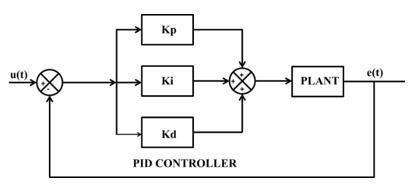Figure 7. PID controller block diagram

4.2.1 Effect on performance specifications

(i) It reduces rise time.

(ii)It increases bandwidth.

(iii) It amplifies noise and hence reduces the signal to noise ratio.

(iv) It increases the damping ratio, ξ and hence reduces peak overshoot.

(v) It eliminates steady-state error between input and output and hence has very high steady-state accuracy.

5. Modes of Operation of Converter Model

5.1 Mode-I operation

## 8.jpgFigure 8. Main circuit model with DC-DC converters during mode-I operation

During this mode of operation, a heavy load is applied to the motor. Due to the load applied, total power is supplied by the UC itself. Switch S3 is in-active state and other two switches S1, S2 are in disable state. This active and OFF states of the switches are decided by the designed controller action only. Here hybrid controller is designed combining PID controller with MFB controller.

5.2 Mode-II operation

In this mode of operation slightly more than the rated load is applied which initiates the operation of the switches. In that switch S3, S1 are both are in–active state, on the other hand, S2 is in disable state. Finally, the total power required by the motor can be supplied by the battery as well as UC. The switches ON and OFF conditions can be decided by the designed hybrid controller which includes PID as well as MFB controller.

## 9.jpg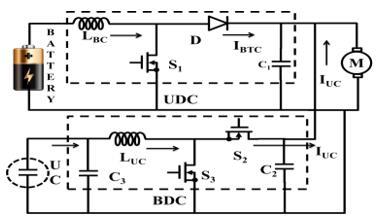Figure 9. Main circuit model with DC-DC converters during mode-II operation

5.3 Mode-III Operation

## 10.jpg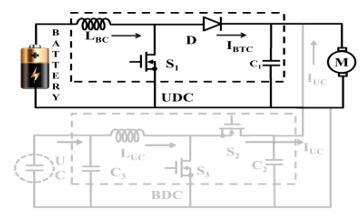Figure 10. Main circuit model with DC-DC converters during mode-III operation

A rated load is applied is applied due to which the switch S1 is only in – active state and other switches S2, S3 are in disable state. In this mode of operation, motor required nominal power which can be supplied by the battery itself. And BDC and UDC operation can be decided by the designed hybrid controller which consists of conventional as well as MFB controller.

5.4 Mode-IV operation

## 11.jpg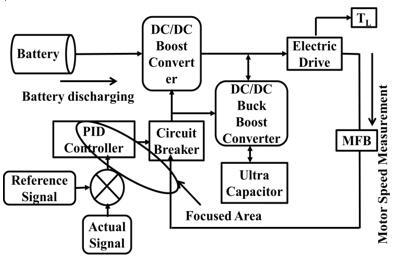Figure 11. Main circuit model with DC-DC converters during mode-IV operation

This mode is related to no load applied to the motor. This initiates the operation of the switches as S1, S2 are in-active state and another switch S3 is in disable state. Which means the battery is supplying total power to the motor as well a UC.

6. Proposed Model Control Strategy

With the proposed control technique production of switching signals to the BDC as well UDC corresponding to the speed of the motor is the main goal of this work. Two controllers are integrated and a hybrid controller for proper selecting of the switches presented in BDC as well as BDC. This technique is implemented to the motor mainly in four modes corresponding to the load applied at a particular instant and those four modes are explained in detail with the flow chart shown in figure 12.

## 12.jpgFigure 12. Flowchart of the proposed control strategy

(1) The MFB controller generates an output signal as 1 for math function U1 and also generates an output signal as 0 for remain math functions U2, U3, U4 corresponding to the speed of an electric motor. In this mode, the motor speed will be ≤ 4800 rpm and the BDC will be in operation which is connected at UC end. Finally, the designed MFB combined with other controller initiates to produce the controlled pulse signals to a particular converter.

(2) If slightly more than rated load is applied to an electric motor due to which motor speed is maintained between 4600 rpm to 4800 rpm. The MBF controller produces output signals as 1 for math functions U1, U2 and generates signals as 0 for remain math functions U3, U4 corresponding to the speed of an electric motor. Finally, controlled signals required by the converters can be generated by the designed MFB combined with another controller. UDC and BDC, both are in the active state, under boost mode. In this mode of operation, UC reduces the burden on the battery by sharing the transient power requirement of the load.

(3) During this mode of operation rated load is applied to the electric motor, which leads to drawing average power by an electric motor. So batteries can delivery total power required by the load. Due to rated load, motor maintains a speed between 4801 rpm to 4930 rpm. The output pulse signal of the designed MFB generates as 1 for math function U3 and generates as 0 for remaining math functions U1, U2, U4 according to the speed of an electric motor. The designed MFB combined with other controller generates a controlled pulse signal to the UDC which will work under boost mode.

(4) During no load or light load condition, the battery is capable to deliver extra power to the load which is used to charge the UC. The output pulse signals of MBF controller generates as 1 for U4 and generates as 0 for U1, U2, and U3 according to the speed of an electric motor. The speed of motor maintained as >4931 rpm. The pulse signals are produced to BDC (buck mode) as well as UDC (boost mode).The converter pulse signal generated to individual switches by the MFB plus PID controller combination, based on the speed of the electric motor explained with bellow fig’s 13, 14 and 15.

## 13.jpgFigure 13. Pulse signals generated structure to switch ONE present in UDC

## 14.jpgFigure 14. Pulse signals generated structure to switch TWO present in BDC

Figure 13 is the structure related to a pulse signal generated to switch S1 based on the speed of the electric motor. Here S1 is related to the UDC, which is connected at the battery end. The pulse signals U2, U3 and U4 generated by the MFB controller are given to the OR gate, which means if the speed of the electric motor is more than 4600 rpm then MFB controller signals are in the active state further this signal can be compared with the signal generated by the conventional controller. Here the conventional PID controller can generate the pulse signal by comparing the reference as well as actual voltage signals of UDC. Finally, the controlled signal of S1 is generated after the successful comparison between the MFB signals as well as the PID controller signal by means of the breaker. In this way, the pulse signal of switch S1 can be controlled based on the speed of the electric motor speed by the Hybrid controller (combination of MFB plus PID). Here the main aim of designed MFB controller is to achieve control action of switches based on the speed of the electric motor for a smooth transition between battery and UC. The designed hybrid controller always works based on the speed of an electric motor for precise switching action between energy sources; this can be taken advantage for proper power splitting between the battery and UC during normal as well transient periods of an electric vehicle.

Figure 14 is the structure related to a pulse signal generated to switch S2 based on the speed of the electric motor. Here S2 is related to the BDC, which is connected at the UC end. The pulse U4 is generated by the MFB controller is given to breaker block directly which means if the speed of the electric motor is more than 4931 rpm then MFB control signal is in the active state, further this signal compared with the pulse signal generated by the conventional PID controller. Here the conventional PID controller generates the pulse signal based on the actual as well as reference voltage signals of BDC. Finally, the controlled signal of switch S2 is generated after comparison between the MFB signal as well as PID controller signal. Here the aim of designed MFB controller is to achieve control action of switches based on the speed of the electric motor for a smooth transition between battery and UC. The designed hybrid controller always works based on the speed of an electric motor for precise switching action between energy sources; this can be taken advantage for proper power splitting between the battery and UC during normal as well transient periods of an electric vehicle.

## 15.jpgFigure 15. Pulse signals generated structure to switch THREE present in BDC

Figure 15 is the structure related to a pulse signal generated to switch S3 based on the speed of the electric motor. Here the switch S3 is related to the BDC, which is connected at the UC end. The pulse signals U1 and U2  are generated by the MFB controller and given to the OR gate, which means if the speed of an electric motor is between 4600 rpm 4800 rpm then MFB control signal will be in the active state, further those signals are compared with the signal generated by the conventional controller. Here the conventional PID controller generates the pulse signals based on the actual as well as reference voltage signals of the BDC. Finally, the comparison has been made between the MFB signal and PID controller signal, after that the controlled signal of switch S3 can be generated. The main aim of the designed MFB controller is to achieve control action of switches based on the speed of the electric motor for a smooth transition between battery and UC. The designed hybrid controller always works based on the speed of an electric motor for precise switching action between energy sources; this can be taken advantage for proper power splitting between the battery and UC during normal as well transient periods of an electric vehicle.

Pulse signal to switch S1: If MFB generates U2 or U3 or U4 then the pulse generated by the PID controller can be applied to switch S1 to initiate the UDC as a boost converter.

Pulse signal to switch S2: If MFB generates only U4 then the pulse generated by the PID controller can be applied to switch S2 to initiate the BDC as a buck converter.

Pulse signal to switch S3: If MFB generates U1 or U2 then the pulse generated by the PID controller can be applied to switch S3 to initiate the BDC as a boost converter.

7. Simulation Results and Discussions

7.1 Mode-I results

## 16.jpg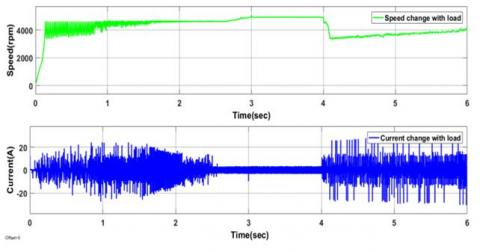Figure 16. Speed and current responses of an electric motor during a heavy load condition

Figure 16 represents that speed variations with the load applied at 4 sec and also current changing according to the load condition. Before applying a load, motor reached the steady state between 2 to 3sec from starting of the motor. During this mode, the heavy load applied to the motor, so that speed reduced to a low value and also huge current variations observed clearly from above Fig 16. During this mode UC only supplies the required power to the motor before motor reaches the steady-state value by PID controller action.

## 17.jpg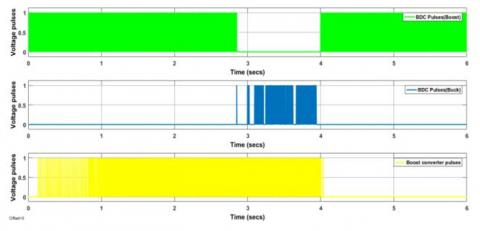Figure 17. Pulse signals generated to converters by the hybrid controller during a heavy load condition

Figure 17 clearly represents the generating pulse signals to the BDC (boost), BDC (buck) and UDC (boost). At starting the motor starts with UC after some time the speed of the motor changes accordingly battery assists the UC and up to 3sec UC and battery together meets the power demand of BLDC and after 3sec to 4sec no-load is applied, during this period UC charges and battery supplies energy to the UC and BLDC. At 4sec, the load is applied and accordingly the speed of the motor reduces, during this particular period UC only meets the power demand of the motor and after some time motor also reaches the steady state by PID controller action.

7.2 Mode-II results

## 18.jpg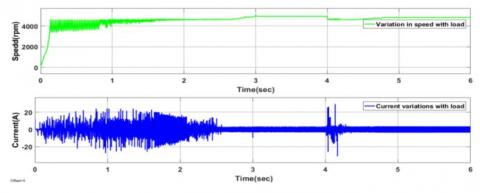Figure 18. Speed and current responses of an electric motor during slightly more than rated load condition

Figure 18 represents the speed variations with the load applied at 4 sec and also current is changing according to the load condition. Before applying a load, the motor has reached the steady state value between 2 to 3sec from starting of the motor. During this mode, normal load is applied to the motor so that speed is reduced to a low value and also huge current variations are observed clearly from Fig. 18. During this mode, UC and battery combine and supply the required power to the motor before motor reaches the steady-state value by PID controller action.

## 19.jpg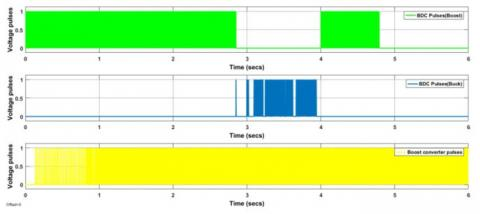Figure 19. Pulse signals generated to converters by the hybrid controller during slightly more than rated load condition

Figure 19 clearly represents that generating pulse signals to the BDC (boost), BDC (buck) and UDC (boost). At starting, motor starts with power from UC, after some time the speed of the motor changes accordingly battery assists UC and up to 3sec UC and battery together meets the power demand of the BLDC and after 3sec to 4sec no-load is applied, during this period battery supplies energy and pulses are also given to BLDC so UC operates in discharging mode to BLDC. At 4sec load is applied on the motor, accordingly the speed of  the motor reduces, during this particular period battery and UC combined meets the power demand of the motor and after some time motor also reaches the steady state speed by PID controller action.

7.3 Mode-III results

Figure 20 represents that speed variations with the load applied at 4 sec and also current changing according to the load condition. Before applying a load, motor reached the steady state between 2 to 3sec from starting of the motor. During this mode, the rated load is applied to the motor so that speed reduced to a low value and also little current variations observed clearly from above Fig 20. During this mode battery only supplies the required power to the motor before motor reaches the steady-state value by PID controller action.

## 20.jpgFigure 20. Speed and current responses of an electric motor during a rated load condition

## 21.jpgFigure 21. Pulse signals generated to converters by the hybrid controller during a rated load condition

Figure 21 clearly represents the generating pulse signals to BDC (boost), BDC (buck) and UDC (boost). At starting, the motor starts with UC, after some time the speed of the motor changes accordingly battery assists the UC and up to 3sec UC and battery together meets the power demand of BLDC and after 3sec to 4sec no load is applied, during this period UC charges and battery supplies energy to UC and BLDC. At 4sec rated load is applied on the motor, accordingly, the speed of the motor reduces, during this particular period battery only meets the power demand of the motor and after some time motor also reaches the steady state speed by PID controller action.

7.4 Mode-IV results

## 222.jpg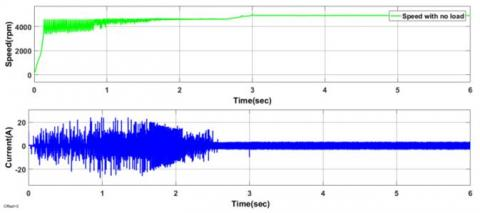Figure 22. Speed and current responses of an electric motor during no load condition

Figure 22 represents that speed variations are applied to load at 4 sec and current is also changing according to the load condition. Before applying a load, the motor has reached the steady state value between 2 to 3sec from starting of the motor. During this mode, the motor is running under no-load so that the speed of the motor never changes and also no changes in current of the motor are observed clearly from Fig 22. During this mode battery only supplies the required power to the motor as well as UC power for charging.

## 23.jpg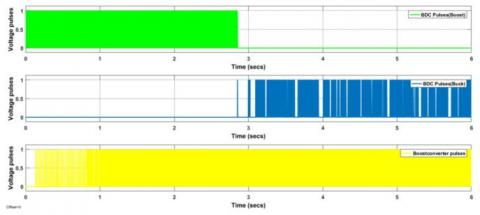Figure 23. Pulse signals generated to converters by the hybrid controller during no load condition

Table 1. DC-DC converters ON/OFF states based on the mode of operation

 S.No Type of mode State of UDC State of BDC Power flow direction 1 Mode-I Off Boost UC supply power to load 2 Mode-II Boost Boost Battery and UC together supply power to Load 3 Mode-III Boost Off Battery only supply power to load 4 Mode-IV Boost Buck The batter can supply power to load as well as UC

Table 2. MFB controller outputs corresponding to speed

 S.No Speed condition associated with mode ON State Math Function 1 If Speed is ≤4800 rpm U1=1&U2=0, U3=0, U4=0 2 If Speed is from 4600 rpm to 4800 rpm U1=1,U2=1& U3=0, U4=0, 3 If Speed is from 4801 rpm to 4930 rpm U3=1 &U1=0, U2=0, U4=0 4 If Speed is >4931 rpm U4=1& U1=0, U2=0, U3=0

Figure 23 clearly represents the generating pulse signals given to BDC (boost), BDC (buck) and UDC (boost). At starting, the motor starts with UC after some time the speed of the motor changes accordingly battery assists the UC and up to 3sec UC and battery together meets the power demand of the BLDC motor. After 3sec no load is applied, during this period UC charges and battery supplies energy to the UC and BLDC.

8. Conclusions

The designed MFB plus PID (Hybrid controller) controller responded satisfactorily to the corresponding output speed of an electric motor for a smooth transition between battery and UC. During a heavy load condition, MFB controller generated a pulse signal as 1 for math function U1; this signal has been compared with conventional PID controller output signal further, initiated the operation of BDC working as a boost converter at UC end. During slightly more than rated load condition, MFB has been generated a pulse signal as 1 at U1 and U2, which can be compared with PID generated signals, further controlled output signals,  starts the operation of the Boost converter at Battery end as well as BDC as a boost converter at UC end. During a rated load condition MFB generated a pulse signal as 1 to U3, which is compared with conventional controller pulses further; this made to operate only UDC as boost converter at the battery end. During no-load operation of the motor, MFB generated a pulse signal as 1 at U4; which again compared with PID generated signal and final generated output initiates the operation of UDC as a boost converter at battery end and BDC as a buck converter at UC end. Finally, the designed hybrid controller worked in four modes and has given satisfactory results. Expect heavy load condition the battery is in ON position and provided the average power. On another hand, UC supplies power during peak power requirements and get charged during the no-load period on the electric motor. All four modes are simulated using MATLAB/Simulink and obtained the satisfactory results and have been plotted and discussed in Simulation results and discussion section.

References

 Tani A, Camara MB, Dakyo B, Azzouz Y. (2013). DC/DC and DC/AC converters control for hybrid electric vehicles energy management-ultracapacitors and fuel cell. IEEE Transactions on Industrial Informatics 9(2): 686-696. https://doi.org/10.1109/TII.2012.2225632

 Wu D, Todd R, Forsyth AJ. (2015). Adaptive rate-limit control for energy storage systems. IEEE Transactions on Industrial Electronics 62(7): 4231-4240. https://doi.org/10.1109/TIE.2014.2385043

 Lustenader EL, Guess RH, Richter E, Turnbull FG. (1977). Development of a hybrid flywheel/battery drive system for electric vehicle applications. IEEE Transactions on Vehicular Technology 26(2): 135-143. https://doi.org/10.1109/T-VT.1977.23670

 Yin H, Zhou W, Li M, Ma C, Zhao C. (2016). An adaptive fuzzy logic-based energy management strategy on battery/ultracapacitor hybrid electric vehicles. IEEE Transactions on Transportation Electrification 2(3): 300-311. https://doi.org/10.1109/TTE.2016.2552721

 Shen J, Khaligh A. (2016). Design and real-time controller implementation for a battery-ultracapacitor hybrid energy storage system. IEEE Transactions on Industrial Informatics 12(5) 1910-1918. https://doi.org/10.1109/TII.2016.2575798

 Wu J, Ruan J, Zhang N, Walker PD. (2018). An optimized real-time energy management strategy for the power-split hybrid electric vehicles. IEEE Transactions on Control Systems Technology, 1-9. https://doi.org/10.1109/TCST.2018.2796551

 Zhang J, Shen T. (2015). Energy management strategy design for plug-in hybrid electric vehicles with continuation/GMRES algorithm. In Control Conference (ECC), 2015 European (pp. 2964-2969). IEEE. https://doi.org/10.1109/ECC.2015.7330988

 Horrein L, Bouscayrol A, Cheng Y, Dumand C. (2015). Hybrid energy management Strategy for hybrid electric vehicle. In Vehicle Power and Propulsion Conference (VPPC), 2015 IEEE, pp. 1-6. https://doi.org/10.1109/VPPC.2015.7352963

 Averbukh M, Lineykin S, Kuperman A. (2015). Portable ultracapacitor-based power source for emergency starting of internal combustion engines. IEEE Transactions on Power Electronics 30(8): 4283-4290. https://doi.org/10.1109/TPEL.2014.2355422

 Xiang C, Wang Y, Hu S, Wang W. (2014). A new topology and control strategy for a hybrid battery-ultracapacitor energy storage system. Energies 7(5): 2874-2896. https://doi.org/10.3390/en7052874